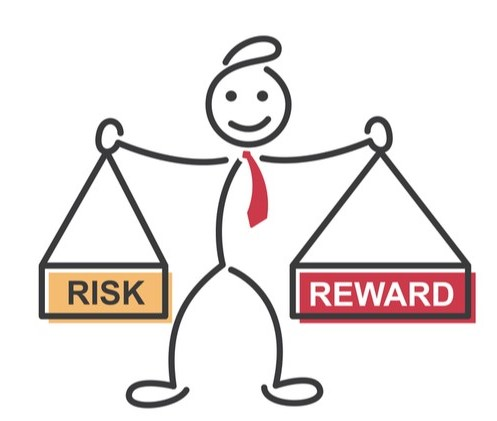## Topic outline

• ### 1. Introduction

Welcome to "Risk, Return, and the Cost of Capital!" The module analyzes the trade-off between risk and return and its implications for the practical estimation of discount rates.##### The main learning goals of this module are:
• Understand the trade-off between risk and return.
• Learn how we can reduce the risk of an asset via diversification.
• Appreciate the basics of modern portfolio theory.
• Get to know the Capital Asset Pricing Model (CAPM) to estimate expected returns.
• See how to obtain the Weighted Average Cost of Capital, the most relevant discount rate in project and firm valuation.
Text and media area: 1 Book: 1
• ### 2. Return and Standard Deviation

This section takes a first look at the relation between risk and return using real market data. This allows us to study the distribution of returns and we get to know the standard deviation of returns, a first very important risk metric. We practice the computation of the standard deviation and conclude with a few words of caution.

Text and media area: 1 Book: 1 Files: 2 Quiz: 1
• ### 3. Introduction to Diversification

This section shows the power of diversification: By picking the appropriate asset, we can reduce the risk of the resulting portfolio without necessarily giving up any return! We present simple examples with only two assets to see diversification at work. We also introduce the concept of "correlation," which tells us to what extent the prices of assets move in unisono.

Text and media area: 1 Book: 1 Quiz: 1
• ### 4. Portfolio with Multiple Assets

This section extends the concept of diversification to portfolios with multiple assets. We get to know the limits of diversification, which is represented by the Efficient Frontier. Then we look at the question of optimal portfolio choice if we can invest in risky as well as risk-free assets. The result is the so-called Capital Market Line, which depicts the best possible portfolios from the point of view of risk and return.  These portfolios offer the maximum possible return for any given level of risk.

Text and media area: 1 Book: 1 File: 1 Quiz: 1
• ### 5. The Capital Asset Pricing Model (CAPM)

Now we are ready to move from portfolio theory to discount rate estimation. This section introduced the Capital Asset Pricing Model (CAPM), which formalizes the relation between the systematic risk and the expected return on an asset. The CAPM is the most popular model to estimate expected returns, which is why it is important to be familiar with it.

Text and media area: 1 Book: 1 Quiz: 1
• ### 6. Estimating the Cost of Capital

Now that we have the necessarily theoretical framework, this module focuses on the practical estimation of expected returns using the CAPM. To this end, we first discuss how to estimate the key ingredients of the CAPM, the risk-free rate, the market risk premium, and the "beta" of the asset in question. Then we discuss the necessary additional steps to obtain the firm's overall expected return, both before and after taxes. The latter is also known as the Weighted Average Cost of Capital (WACC) and constitutes the most important discount rate in firm and project valuation.

Text and media areas: 2 Book: 1 Quiz: 1 URL: 1
• ### 7. Practical Implementation

We conclude the module with a simple check-list and a practical example. The check-list shows how to proceed when we need to estimate the cost of capital (WACC) of a project for which we cannot directly estimate the necessary risk parameters. Finally, we use this check-list to determine the WACC of an unlisted software company in Germany.

Text and media area: 1 Book: 1 Quiz: 1